# Effects of mesh loop modes on performance of unstructured finite volume GPU simulations

## Abstract

In unstructured finite volume method, loop on different mesh components such as cells, faces, nodes, etc is used widely for the traversal of data. Mesh loop results in direct or indirect data access that affects data locality significantly. By loop on mesh, many threads accessing the same data lead to data dependence. Both data locality and data dependence play an important part in the performance of GPU simulations. For optimizing a GPU-accelerated unstructured finite volume Computational Fluid Dynamics (CFD) program, the performance of hot spots under different loops on cells, faces, and nodes is evaluated on Nvidia Tesla V100 and K80. Numerical tests under different mesh scales show that the effects of mesh loop modes are different on data locality and data dependence. Specifically, face loop makes the best data locality, so long as access to face data exists in kernels. Cell loop brings the smallest overheads due to non-coalescing data access, when both cell and node data are used in computing without face data. Cell loop owns the best performance in the condition that only indirect access of cell data exists in kernels. Atomic operations reduced the performance of kernels largely in K80, which is not obvious on V100. With the suitable mesh loop mode in all kernels, the overall performance of GPU simulations can be increased by 15%-20%. Finally, the program on a single GPU V100 can achieve maximum 21.7 and average 14.1 speed up compared with 28 MPI tasks on two Intel CPUs Xeon Gold 6132.

## 1 Introduction

CFD plays an important part in the design of aircraft , which is applied in many scenarios including predicting aerodynamics of wing , analyzing combustion in engine , and so on. More and more computing resources are required, for high precision analysis. For example, high resolution mesh should be used in Large Eddy Simulation (LES)  and Direct Numerical Simulation (DNS) . Thus, many CFD simulations  must be performed in high performance computing (HPC) systems from clusters to supercomputers. With the development of computing technology, the General Purpose Graphics Processing Unit (GPGPU)  has played a more and more important role in HPC system , due to low energy consumption and high computing efficiency. Many mesh based CFD codes  have been ported and optimized on GPU.

Unstructured mesh is used widely in CFD for aeronautics and astronautics applications. That’s because the construction of unstructured mesh is much easier for the computational domain with complex geometry  owned by aircraft. Furthermore, unstructured mesh is adopted by many CFD software or computational frameworks including FUN3D , OpenFOAM , SU2 , Fluidity , NNW-PHengLEI , and so on. However, compared with GPU simulations on structured mesh , it is difficult to get high computational performance by unstructured mesh-based GPU simulations, due to severe data locality problem .

Data locality affects the performance significantly of GPU computing. Specifically, on the unstructured mesh, data storage is irregular. Indirect data access results in non-coalescing load or store of data on device memory. Overheads due to non-coalescing data load and storage are aggravated by multi-thread access data mode on GPU . High memory bandwidth can be obtained in some compact algorithms such as high-order Discontinuous Galerkin (DG)  methods and Flux Reconstruction (FR) . However, it is still difficult to improve the data locality of the second-order Finite Volume (FV)  method. In order to reduce the overheads of memory indirect access, reorder of cell index or face index can be used . However, for complex geometry, the effects of reordering are weak . Some researchers studied the influence of SOA and AOS data layout on data locality . However, indirect memory access still exists in different data layouts. In fact, data array index sorting can be adjusted by different mesh loops. It is significant to investigate the effects of mesh loop modes on data locality of GPU computing.

Data dependence also influences the performance remarkably on GPU. The race condition is often induced by multi-threading updating the same global memory or shared memory, which can be resolved by hardware based method or software based method. Thread lock on GPU can be used to make sure that data is only updated by one thread. The overheads of thread lock have been reduced since Nvidia Kepler architecture . Graph coloring method  can also be applied to guarantee that data in one color group is accessed by different threads. It is found that thread lock is more efficient than graph coloring method  on Nvidia Tesla V100. By adjusting mesh loop mode, race conditions can be eliminated as well.

In this paper, the effects of different mesh loops on GPU computing are investigated. Several GPU kernels are studied in a second-order finite volume CFD solver. Those GPU kernels are all hot spots, which account for more than 70% executing time on both GPU Tesla V100 and K80. Furthermore, only face loop is used in those kernels. Considering both data locality and dependence, the performances of those hot spots are evaluated and analyzed for different mesh loop modes. Specifically, according to performance comparison, it is found that in the kernel for interpolation data from cells to nodes, cell loop can achieve the best performance comparing with face loop and node loop. Besides interpolation, mesh loop modes for local maximum & minimum pressure are studied as well. It also indicates that cell loop is more efficient than face loop. The remaining paper is organized as follows: Section 2 introduces the mathematical model and mesh loop modes. Section 3 gives algorithms under different mesh loops and is followed by Section 4 for performance optimization and analysis. Finally, the paper presents conclusions and future work in Section 5.

## 2 Mathematical model

### 2.1 Governing equations and finite volume discretization

PHengLEI has been developed by China Aerodynamics Research and Development Center  for simulating high speed and compressible flow. The governing equations are Navier-Stokes (NS) Equations described in a small three-dimensional control volume Ω with boundary dΩ, shown by Eq. 1,

$$\frac{\partial }{{\partial t}}\int_{\Omega} {\vec q} d\Omega + \oint_{\partial \Omega } {\left({{{\vec F}_{c}} - {{\vec F}_{v} }} \right)} dS = 0$$
(1)

where $$\vec q$$ containing 5 unknowns are described in Eq. 2, including density ρ, velocity components u, v, w, and internal energy e.

$$\vec F_{c}$$ and $$\vec F_{v}$$ are convective flux and viscous flux respectively, described in Eq. 3 and Eq. 4. Specifically, $$\vec F_{c}$$ is related with 5 unknowns and V on dΩ. V is obtained by V=nxu+nyv+nzw. nx,ny, and nz are components of normal vector on dΩ. $$\vec F_{v}$$ is related with normal vector, viscous stress tensor with components from τxx to τzz, and energy dissipation terms including θx,θy, and θz. Both viscous stress and energy dissipation terms are related with gradient of $$\vec q$$ and some physical coefficients on dΩ.

$$\vec Q = \left[ {\begin{array}{c} \rho \\ {\rho u}\\ {\rho v}\\ {\rho w}\\ {\rho e} \end{array}} \right]$$
(2)
$${\vec F}_{c} = \left[ {\begin{array}{c} \rho V \\ {\rho uV+n_{x}p}\\ {\rho vV+n_{y}p}\\ {\rho wV+n_{z}p}\\ {\rho V(e+\frac{u^{2}+v^{2}+w^{2}}{2}+\frac{p}{\rho})} \end{array}} \right]$$
(3)
$${\vec F}_{v} = \left[ {\begin{array}{c} 0 \\ {n_{x}\tau_{xx}+n_{y}\tau_{xy}+n_{z}\tau_{xz}}\\ {n_{x}\tau_{yx}+n_{y}\tau_{yy}+n_{z}\tau_{yz}}\\ {n_{x}\tau_{zx}+n_{y}\tau_{zy}+n_{z}\tau_{zz}}\\ {n_{x}\theta_{x}+n_{y}\theta_{y}+n_{z}\theta_{z}} \end{array}} \right]$$
(4)

In the cell-centered finite volume method, the physical domain is discretized into a number of control volumes (also called cells). The boundary of volumes is called faces. If a particular cell Ωvol owns NF faces with area Sm, NS equations can be discretized as:

$$\frac{{\Delta{{\vec q}_{vol}}}}{\Delta{t}} = - \frac{1}{{{\Omega_{vol}}}}\left[ {\sum\limits_{m = 1}^{{N_{F}}} {{{\left({{{\vec F}_{c}} - {{\vec F}_{v} }} \right)}_{m}}{S_{m}}} } \right]$$
(5)

The calculation of both $$\vec F_{c}$$ and $$\vec F_{v}$$ requires gradient of $$\vec q_{vol}$$ with components including $$\frac {\partial \vec q_{vol}}{\partial x}, \frac {\partial \vec q_{vol}}{\partial y}$$, and $$\frac {\partial \vec q_{vol}}{\partial z}$$. The gradient of $$\vec q_{vol}$$ can be obtained by Gauss-Green theory, as

$${\begin{array}{c} \frac{\partial \vec q_{vol}}{\partial x}=\frac{1}{\Omega_{vol}}\left[ {\sum\limits_{m = 1}^{{N_{F}}} {{{\left(\frac{1}{N_{N}}{\sum\limits_{n = 1}^{{N_{N}}}{\vec q_{n}}} \right)}_{m}{n_{x}}}{S_{m}}} } \right] \\ \frac{\partial \vec q_{vol}}{\partial y}=\frac{1}{\Omega_{vol}}\left[ {\sum\limits_{m = 1}^{{N_{F}}} {{{\left(\frac{1}{N_{N}}{\sum\limits_{n = 1}^{{N_{N}}}{\vec q_{n}}} \right)}_{m}{n_{y}}}{S_{m}}} } \right] \\ \frac{\partial \vec q_{vol}}{\partial z}=\frac{1}{\Omega_{vol}}\left[ {\sum\limits_{m = 1}^{{N_{F}}} {{{\left(\frac{1}{N_{N}}{\sum\limits_{n = 1}^{{N_{N}}}{\vec q_{n}}} \right)}_{m}{n_{z}}}{S_{m}}} } \right] \\ \end{array}}$$
(6)

where $$\vec q_{n}$$ is an unknown independent vector on faces’ nodes and NN is the total number of nodes on a face.

By the average of $$\vec q_{vol}$$ on total NC cells that share the same node, $$\vec q_{n}$$ on the node is obtained by

$$\vec q_{n} = \frac{1}{{{N_{C}}}}\left[ {\sum\limits_{c = 1}^{{N_{C}}} {{{\left({\vec q_{vol}} \right)}_{c}}} } \right]$$
(7)

The reconstruction of $${\vec q}_{vol}$$ from cell center to faces is required for computing $$\vec F_{c}$$.The reconstruction term $$\vec q_{m}$$ can be described by $$\vec q_{m}=\vec q_{vol}+\phi (\frac {\partial \vec q_{vol}}{\partial x} l_{mx}+ \frac {\partial \vec q_{vol}}{\partial y} l_{my}+\frac {\partial \vec q_{vol}}{\partial z} l_{mz})$$. lmx,lmy, and lmz are components of vector from cell center to face center. ϕ is the limiter to avoid physical oscillation, which is described by

{\begin{aligned} \phi &= \frac{1}{\eta_{-}} \left[{\frac{{\eta_{+}}^{2}\eta_{-} + 2{\eta_{-}}^{2}\eta_{+}}{{\eta_{+}}^{2}+2{\eta_{+}}{\eta_{-}}+{\eta_{-}}^{2}}}\right]\\ \eta_{+} &= \left\{\begin{array}{l} p_{vol}^{max} - p_{vol}\\ p_{vol}^{min} - p_{vol} \end{array}\right.\\ \eta_{-} &=\frac{ \partial \vec q_{vol}}{\partial x} l_{mx}+ \frac{\partial \vec q_{vol}}{\partial y} l_{my}+\frac{\partial \vec q_{vol}}{\partial z} l_{mz} \end{aligned}}
(8)

where pvol is one component of $$\vec q_{vol}$$, which is the pressure located on the cell center. $$p_{vol}^{max}$$ and $$p_{vol}^{min}$$ are the local maximum and minimum pressure on the volume.

The convective flux $$\vec F_{c}$$ is calculated by the Roe scheme and the viscous flux $$\vec F_{v}$$ is discretized by the central scheme. The computing process of the turbulence model is similar to NS equations, which are not described in detail. Spalart-Allmaras turbulence model is applied for turbulence effects. Iteration is required to guarantee that residuals of NS equations and the turbulence equation on each cell are small enough. In every iteration step, the explicit two order Runge Kutta method is applied to discretize the unsteady term in Eq. 5.

### 2.2 Mesh and data storage

In the Finite Volume method, the computing domain must be discretized in advance. Mesh is formed by a number of non-overlapping arbitrary polyhedral control volumes that fulfill the computing domain, shown in Fig. 1a. The boundary of control volume (cell) is called faces. The connection of the face edge is called nodes. Three-dimensional mesh’s cells, faces, and nodes are shown in Fig. 1b. Here, for simplification, hexahedral cells are used for describing mesh loop modes. In fact, most cells in Fig. 1a are tetrahedral. Each cell, face, and node have their own unique index. Due to unstructured property, cells, faces, or nodes with adjacent indexes do not mean connectivity in the physical domain. Hence, physical connectivity between cells, faces, and nodes is defined by index reflection.

In order to better explain the data structure and storage method, we stipulate that the name of the scalar will be in normal style, and the name of the array will be in italics.

Face-node connectivity is defined by faceNodes and nodeFaces. faceNodes is a one-dimensional array which focuses on faces and stores the nodes they connect with. As shown in Fig. 1b, face fi has four nodes including ne,nf,ng and nh (indexes order: ne<nf<ng<nh), which means the numNodesInFace of fi is 4. The nodes’ indexes will be stored in faceNodes through ascending order. For example, faceNodes[nodeOfFaceStart[ fi]]= ne, faceNodes[nodeOfFaceStart[ fi]+1]= nf, where the nodeOfFaceStart is an array that points out the index offset for a face. On the contrary, the nodeFaces stores the connective faces of different nodes in a similar manner, with the help of numFacesInNode and faceOfNodeStart.

Cell-face connectivity is defined by owner, neighbor, and cellFaces. Cell index is stored in owner and neighbor, by the order of face index. The cell with smaller index sharing the same face is stored in owner, while the larger one is stored in neighbor. In Fig. 1b, cell O and cell P (O<P) share the same face fi. Their face-cell connectivities can be described by owner[ fi]=O and neighbor[ fi]=P. Similarly, face indexes are stored in cellFaces, equipped with numFacesInCell and faceOfCellStart.

Cell-node connectivity is defined by cellNodes and nodeCells in a similar manner as described in the face-node connectivity.

Based on the mesh, Eq. 1 can be approximately changed into Eq. 5 through numerical methods. Apart from cell data q, the surface integral in Eq. 5 contains fluxes ($${\vec F}_{c}$$ and $${\vec F}_{v}$$) crossing faces of control volumes. Those fluxes are stored on face centers. In the computing of face data flux, many data on faces are needed. Besides cell data and face data, qNode stored on nodes of mesh is also required by Eq. 6. As a result, the access of cell data, face data and node data is performance critical for an unstructured finite volume mesh (see Fig. 1b).

Structure of Array (SOA) data layout is used for cell data, face data, and node data in host memory and device memory. Thus, cell data, face data, and node data can be accessed directly by cell index, face index, and node index respectively. Because of the topological connectivity definition, the indirect data accessed problem cannot be avoided in some kernels that are computed with cell, face and node data. For example, node data can be accessed indirectly based on the faceNodes, numNodesInFace, and nodeOfFaceStart.

### 2.3 Mesh loop modes

In the unstructured finite volume CFD program, a loop on mesh components is used for the traversal of data located on the mesh. With different data types, direct or indirect data access can be induced by different mesh loop modes. Obviously, data on cells, faces, and nodes can be directly accessed by the corresponding loop on cells, faces, and nodes respectively. However, more than one type of data is used in most kernels, which induces indirect data access problem in mesh loop.

Taking the gradient of q for instance (the part in square brackets of Eq. 6), where cell data, face data, and node data all exist in computing. In PHengLEI code (CPU version), face loop from index 0 to numTotalFaces-1 is applied in Algorithm 1. Thus, face data including normal vector (nxs, nys, nzs) and area area are loaded directly by face index (Line 11-14). On the contrary, the gradient of q including dqdx, dqdy, and dqdz located on cells is stored indirectly due to the cell-face connectivity information like owner and neighbor (Line 15-20).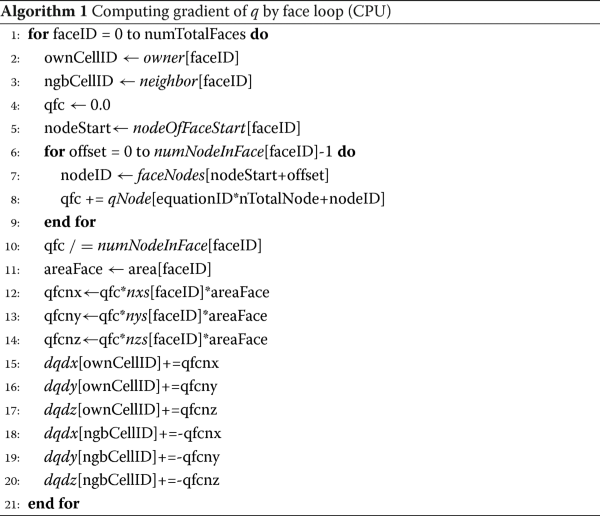The face loop for the gradient of q is ported to GPU, described in Algorithm 5. It should be noted that all of the data used in GPU is allocated in global memory, with the same name used in CPU. The indirect access of cell data and node data by face loop leads to non-coalescing global memory load and store which brings great latency troubles.

Besides face loop, there are two more loop modes, cell loop and node loop, which can be applied for computing gradient of q (described in Algorithms 6 and 7). Although the indirect data access problem is inevitable in different mesh loops, the loop with better data locality and less latency overhead should be considered firstly for performance optimization.

Besides data locality, it can be observed that, in Algorithm 5 (Line 16-21) and Algorithm 7 (Line 14-19), atomic operations are used for resolving race condition. To illustrate, in both node loop and face loop, several threads may update the same cell data. The data dependence should be guaranteed by atomic operations. Overheads induced by those atomic operations should be put into consideration as well.

### 2.4 Hot spot analysis

Most functions in the unstructured FVM CFD program PHengLEI have been already ported on GPU V100. The mesh loop modes used in GPU kernels have the same implement in corresponding CPU functions. Through profiling , there are 6 kernels that account for around 75% of executing time in total, as mesh scale varies from 1 million to 9 million. Those kernels’ function and data type are described in Table 1. The execution time proportion of 6 hot spots (GPU kernels) is shown in Table 2. Furthermore, the speedup of those kernels running on a single GPU compared with CPU code on one core is described in Table 3. The speedup of 4 kernels including GPUGradientQ, GPUNodeValue, GPUFluxSum and GPULocalMinMax is always smaller than the average value in different mesh scales. Hence, it is significant to optimize those 4 kernels in the next stage.

In Table 1, face loop is used in all hot spots kernels. However, not all the kernel contains face data, for example, GPUNodeValue only uses cell data and node data. Face loop may aggravate non-coalescing of data access. Therefore, the performance of different mesh loop modes should be investigated and the suitable mesh loop mode should be applied.

## 3 Algorithms for different mesh loop modes

Algorithms for four computing procedures including interpolating and gradient of q, sum of flux, local maximum and minimum pressure determination are described in this section. For each procedure, GPU kernels by face loop will be introduced, while the face loop or node loop will be proposed depending on the data type.

### 3.1 Interpolating q

Interpolating q from cells to nodes is described in Eq. 7. The computing can be ported to GPU under different loop modes.

Algorithm 2 (NV-F) shows the interpolation of q by face loop on GPU. For each face, qNode is located on nodes to be accessed. Cell data q on both face’s owner and neighbor cells is added into qNode. Similarly, cell data t is added into tNode. Node data nCount gets how many cells are contributed to each node. Atomic operation is used to avoid race conditions that may be induced by updating qNode on the same node belonging to faces computed by different threads.Algorithm 3 (NV-C) describes the kernel by cell loop mode for interpolation of q. GPU threads are assigned according to cells. In one cell, the traversal of nodes is performed so that cell data q and t can be added into qNode and tNode respectively. Atomic operation is also necessary to resolve race conditions.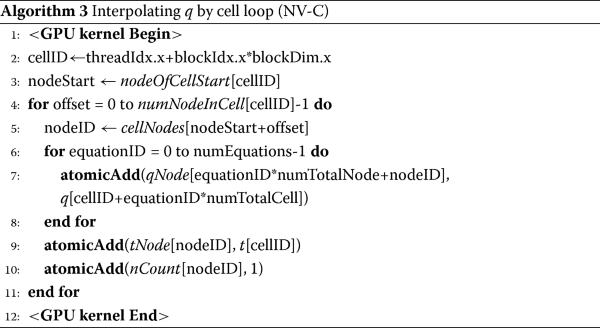Algorithm 4 (NV-N) introduces the use of node loop for interpolation of q. GPU threads’ calculation is based on nodes. For each node, the traversal of cells that own the same node is done for adding cell data q into qNode. Race conditions can be avoided by the node loop mode. Atomic operation is not necessary.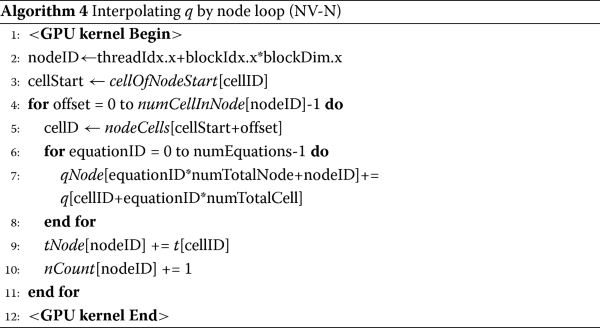The gradient of q is computed according to Eq. 6. As Ωvol is a constant value in each cell, the part in square brackets of Eq. 6 should be paid more attention. GPU kernels with different loop modes implement can achieve the same requirement.

Algorithm 5 (GQ-F) describes the GPU kernel for the gradient of q by face loop. For each face, the average of qNode on nodes is stored in qfc temporarily. Then, face data including area area and normal vector (nxs, nys, nzs) are multiplied with qfc. Finally, temporary face variables including qfcnx, qfcny, and qfcnz are added into dqdx, dqdy, and dqdz respectively on both neighbor and owner cell. Race conditions may be induced as several GPU threads write into the same cell data. Therefore, the atomic operation is required.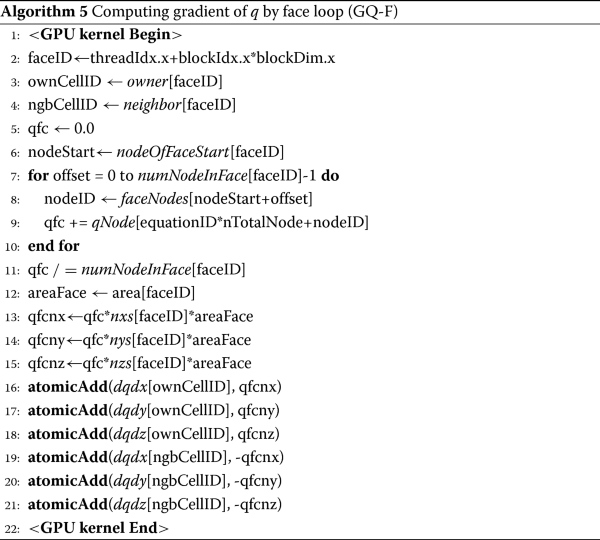Algorithm 6 (GQ-C) expounds the application of cell loop on gradient of q. GPU threads are assigned to cells. As to a cell, the traversal of faces is performed to get the face data including area, nxs, nys, and, nzs. For each face, node data qNode on the face’s nodes is loaded for the average qfc. Finally, cell data including dqdx, dqdy, and dqdz are stored by temporary face data including qfcnx, qfcny, and qfcnz. The contributions of faces to owner cell and neighbor cell are distinguished by leftRightFace.Algorithm 7 (GQ-N) indicates how the node loop is used for the gradient of q in a GPU kernel. For each node, the node data qNode is loaded for the average qfc. The traversal of faces that own the same node is performed to get the face data including area and normal vector. The atomic operation should be used for adding qfcnx, qfcny, and qfcnz to dqdx, dqdy, and dqdz respectively, because many threads may write to the same cell data.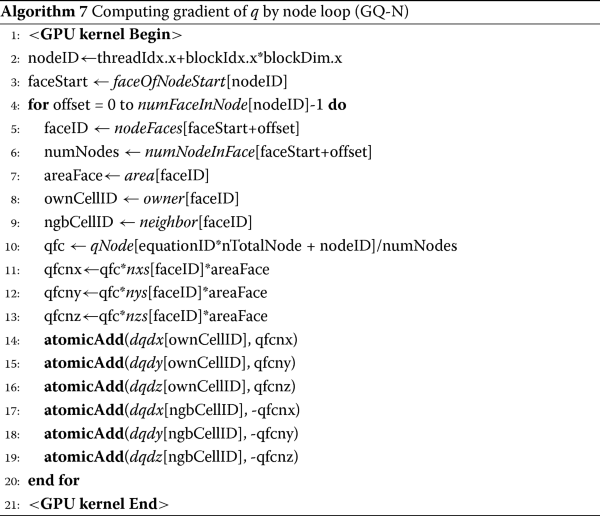### 3.3 Summation of flux

In Eq. 5, summation of flux res on faces of each cell is required for computing convective flux $$\vec F_{c}$$ and viscous flux $$\vec F_{v}$$. Both face loop and cell loop can be applied.

Algorithm 8 (SF-F) describes summation of flux by face loop on GPU. On a face, flux located on faces is loaded. Then, flux is added to res on both the owner cell and neighbor cell.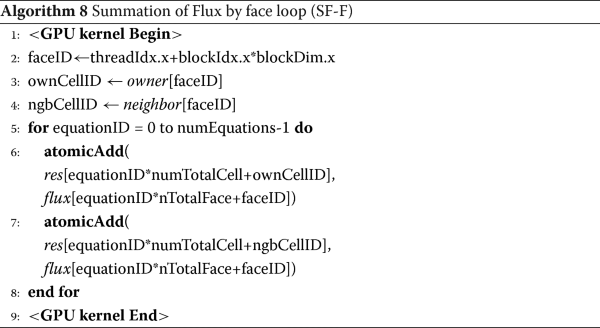Algorithm 9 (SF-C) shows the application of cell loop for summation of flux. GPU threads are assigned to cells. In a cell, face data flux is loaded by a traversal of faces. Then, flux is added to the cell data res. ownNgbFace is used for distinguishing neighbor or owner cell sharing the same face.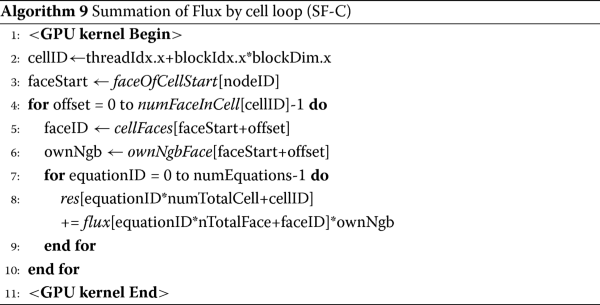### 3.4 Computing local maximum and minimum pressure

Local maximum and minimum pressure $$p_{vol}^{max}$$ and $$p_{vol}^{min}$$ in Eq. 8 are computed by comparing pressure pvol on one cell with pressure on the cell’s neighbor cells.

Algorithm 10 (LM-F) describes the determination of local maximum and minimum pressure by face loop. For each face, by comparing pressure and dMin on cells sharing the same face, the smaller one is stored into dMin. Similarly, comparing pressure and dMax on cells sharing the same face, the larger one is stored into dMax. Finally, the traversal of faces makes pressure on each cell compared with the surrounding neighbor cells.Algorithm 11 (LM-C) shows how to obtain each cell’s local maximum and minimum pressure by cell loop. For each cell, by looping on its boundary faces, pressure on the cell’s surrounding neighbor cells can be loaded and compared with that on the cell. After the comparison, the local maximum and minimum pressure is stored into dMax and dMin on the cell, respectively.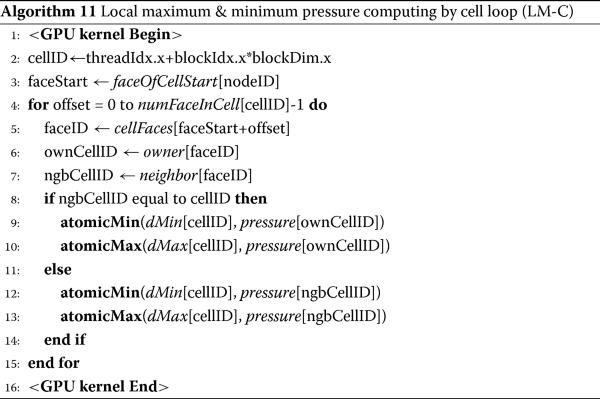## 4 Performance analysis

### 4.1 Performance test and computing environment

The performance benchmark is the simulation of transonic flow over an ONERA M6 wing. Unstructured mesh is used in our evaluations. The three-dimensional computational domain is filled by hexahedral and tetrahedral hybrid cells, shown in Fig. 1a. The topology connectivity in the mesh is general in CFD so that the results of the performance test are generalized for unstructured CFD applications. Five meshes used in performance evaluation are described in Table 4. 9 million mesh is used in numerical experiments, which is close to the maximum mesh scale computed by PHengLEI GPU version on a single GPU V100.

All of the algorithms in Section 3 are implemented by CUDA C language. Both Nvidia Tesla K80 and V100 are used in performance tests for investigating the influence of Kepler architecture and Volta architecture. CUDA 8.0 and CUDA 10.0 are used as drivers for K80 and V100, respectively. Every GPU kernel is repeated 100 times on a single GPU and the total executing time is recorded in Table 5 and Table 6.

### 4.2 Validation of GPU simulations

For validating GPU simulations, an ONERA M6 in a transonic uniform flow is simulated by GPU and CPU respectively. Specifically, the inflow velocity is set by Mach number of 0.8395 at the attack angle of 3.06 rad and the sideslip angle of 0 deg. Firstly, the aerodynamic coefficients including drag coefficient and lift coefficient are compared between GPU and CPU. Figure 2a and b show that the difference of aerodynamic coefficients between CPU and GPU is very small. For evaluating the difference of aerodynamic coefficients between CPU and GPU, it is defined by error=CgpuCcpu. From Fig. 2c and d, it can be seen that the error is around 10−12 during 2×105 iteration steps. Compared with the order of aerodynamic coefficients, the small error can be ignored. Secondly, the pressure coefficient (Cp) contour on the wing surface is also compared between GPU and CPU. Figure 3 shows that the difference of pressure coefficient is subtle on both front and back surfaces. The validation indicates that GPU simulation results are precise with guarantee.

### 4.3 Interpolation of q

Face loop, cell loop, and node loop are used in kernels NV-F, NV-C, and NV-N respectively, for computing the interpolation of q. In those mesh loop modes, data locality is completely different. Specifically, the load of cell data is only coalescing in the cell loop. Face loop and node loop make the access of cell data indirect, which leads to much more overheads. Similarly, the store of node data is coalesced only in the node loop. Face loop and cell loop induce indirect access of node data, which aggravates the latency of updating global memory. Besides data locality, atomic operations are necessary for the face loop and cell loop. The implicit synchronization induced by atomic operations may reduce the performance. Hence, the effects of different mesh loop modes on the interpolation of q should be shown in data locality and atomic operations.

The executing time of NV-F, NV-C, and NV-N on V100 and K80 is all normalized by that of NV-F. The comparison of performance is shown in Fig. 4. It indicates that on both V100 and K80, the face loop in NV-F gets the worst performance. That’s because the face loop makes both node data and cell data accessed indirectly, which leads to the terrible latency. Furthermore, atomic operations are also one of the causes of poor results.

On V100, the cell loop consumes a smaller executing time than the node loop from Mesh 1 to Mesh 4. The performance gap between the cell loop and node loop goes down with the increase of mesh size. On the largest mesh (Mesh 5 with 8.78 million cells and 3.50 million nodes), the node loop is even better than the cell loop. On the contrary, on K80, the performance of the node loop is always better than that of the cell loop from Mesh 1 to Mesh 5. Considering the same topology information in mesh, overheads due to data locality should be similar. Hence, the reverse performance on V100 and K80 may be related to atomic operations. Effects of atomic operations on cell loop should be further investigated.

Cell loop without atomic operations is applied based on kernel NV-C. It is easy to directly get rid of atomic operations in Algorithm 3, regardless of the simulation results. The executing time of the cell loop without atomic operations on V100 and K80 is shown in Table. 7. Normalized by cell loop with atomic operations, the comparison of cell loop with and without atomic operations is shown in Fig. 5. It is found that atomic operations bring negative effects on K80 while the influence is not significant on V100. Therefore, on K80, latency due to atomic operations makes the cell loop worse than the node loop. On V100, most overheads are induced by data locality, which makes the cell loop better.

Face loop, cell loop, and node loop can be used for the gradient of q in GPU kernels GQ-F, GQ-C, and GQ-N, respectively. In gradient of q, face data, cell data, and node data are all used. Hence, one loop mode can guarantee only one type of data to be accessed directly, as the other two types of data are accessed indirectly. Besides, due to the store of cell data, atomic operations are required in both face loop and node loop, and data locality and atomic operations should be considered simultaneously for the performance in different loop modes.

In order to compare the performance of different loop modes, the executing time of face loop is used as a denominator for normalizing. The normalized executing time is shown in Fig. 6.

Compared with face loop, node loop is more than 10 times and 15 times slower on K80 and V100 respectively. So, the overheads of non-coalescing access to cell data and face data are the most significant by node loop. Cell loop consumes more than 2 times executing time on V100 and around 1.5 times on K80 than face loop. It means that although atomic operations can be avoided in cell loop, latency from indirectly accessing face and node data is still much more serious than node loop.

### 4.5 Summation of flux

In summation of flux, both cell data and face data can be used. Face loop and cell loop are applied in GPU kernels SF-F and SF-C, respectively. In face loop, cell data is accessed indirectly. Furthermore, atomic operations are required to address race conditions. In cell loop, indirect access to face data exists. Thus, performance is affected by indirect data access and atomic operations in different loop modes.

To compare performance between face loop and cell loop, executing time of GPU kernels is normalized by that of SF-F as shown in Fig. 7.

On V100, face loop outperforms cell loop from Mesh 1 to Mesh 4. Though atomic operations are not used in cell loop, the performance of face loop is better. Thus, overheads due to non-coalescing access of face data in cell loop are much larger than that induced by cell data indirect access in face loop. The performance gap between face loop and cell loop decreases with the increase of mesh size. Even on Mesh 5 with 8.78 million cells and 20.68 million faces, face loop consumes a little more executing time than cell loop.

On K80, cell loop’s performance is much better from Mesh 1 to Mesh 5. Considering that the overheads due to non-coalescing in face loop are much less than that induced by cell loop, atomic operations in face loop must make much more overheads. It shows again that atomic operations make performance descend remarkably on K80.

### 4.6 Determination of local min and max pressure

In the calculation of local min and max pressure, only cell data is used. Face loop is used in CPU code. Both face loop and cell loop are considered in GPU kernels LM-F and LM-C, respectively. In face loop, all of cell data is accessed indirectly. Furthermore, atomic operations are required for resolving multi-thread on faces updating the same cell data. On the other hand, in cell loop, dMin and dMax can be updated directly without atomic operations. Indirect access to pressure still exists on each cell’s neighbor cells.

For comparing performance between face loop and cell loop, executing time of LM-F is used for normalizing GPU kernels, described in Fig. 8.

It can be seen that on both V100 and K80, cell loop is more efficient than face loop. It means that overheads due to non-coalescing data access by face loop are more than those by indirect access to cell’s neighbor cell. On K80, cell loop’s execution time is less than 0.7 of face loop’s, compared with the time ratio close to 0.8 on V100. Obviously, atomic operations make face loop’s performance even worse on K80.

### 4.7 Which loop mode should be used?

From the comparison of different mesh loop modes, it can be seen that mesh loop modes affected GPU kernels’ performance significantly by data locality and data dependence (race condition). The suitable mesh loop mode should have low latency of non-coalescing global memory access and no race condition. However, in most conditions, the choice of mesh loop depends on the trade-off between data locality and data dependence.

If only one type of data is used, the same type mesh loop mode should be used for better data locality without a race condition. Take determination of local min and max pressure for example, cell loop can make some cell data access coalesced and smaller overheads induced by indirect access of neighbor cells’ data.

Face loop should better be used for the best data locality, as face data exist in computing. Performance analysis of gradient of q and summation of flux in different mesh modes show that overheads by face loop indirectly accessing cell data or node data are the smallest.

When both cell data and node data are applied without face data, cell loop can gain good data locality. Interpolation of q shows that overheads induced by cell loop indirect access of node and cell data are the smallest.

Atomic operation’s consumption is significant on K80. The comparison of interpolating q by cell loop with and without atomic operations shows that atomic operations make performance reduce remarkably. On the contrary, atomic operations’ influence is little on V100. Therefore, after Kepler’s architecture, data locality should be considered for performance firstly. It is shown by summation of flux, face loop with atomic operations is superior to cell loop with no race condition on V100.

### 4.8 The overall performance after optimization

All of the computing produces are offloaded on GPU. Data transfer between host and device is only performed before and after the main time loop of simulation. The other GPU kernels that are not discussed in this paper are also adjusted according to the principles proposed above. Besides optimization of data transfer and loop modes, the consumed time for creation and destruction of temporary variables in iterations is also reduced remarkably. Those temporary variables are replaced by global variables that are just created and destroyed once in the simulation.

The computing platform owns 4 GPUs (V100) and 2 CPUs (Intel Xeon Gold 6132). Each CPU contains 14 cores so that 28 CPU cores can be used in the computing platform. Executing time is recorded from the 100th iteration step to the 300th iteration step. The ratio (speedup) of executing time between 28-MPI CPU simulation and 1 GPU simulation is shown in Table 8 on different mesh scales. It indicates that average speedup of 14.1 and maximum speedup of 21.7 can be achieved.

## 5 Conclusion and future works

In this paper, we apply different mesh loop modes on 4 hot spots of GPU kernels in the unstructured FVM CFD program PHengLEI. The performances of those kernels are evaluated under 5 different mesh scales. The performance analysis shows that mesh loop modes affect kernels’ performance significantly through data locality and data dependence. It is concluded that as access to face data is required in kernels, face loop results in the smallest overheads due to non-coalescing access, compared with cell loop and node loop. Performance of gradient operation shows that face loop only consumes 1/2 time of cell loop and 1/10 of node loop. Cell loop achieves the best data locality, when both cell data and node data are accessed, but face data do not exist in kernels. Cell loop makes the best performance, as non-data coalescing access is only induced by cell data. On GPU K80, atomic operations induced remarkable overheads so that cell loop outperforms face loop, though face loop owns better data locality. On GPU V100, the effects of atomic operations are not obvious. In interpolation of data from cells to nodes, cell loop consumes less time than node loop on V100. On the contrary, on K80, executing time of cell loop is a little larger than that of face loop. Thus, it should be paid more attention to mesh loop modes. Optimized by suitable mesh loop mode, the overall GPU V100 accelerated CFD program can achieve maximum 21.7 (average 14.1) speedups vs. 28 MPI CPU implement.

In future work, reducing the overhead of indirect data assessment and data dependence will be the principal target in research. More GPU architectures such as Nvidia A100, AMD series GPU will be taken into consideration to investigate the effects of different loop modes and discover architecture features that are beneficial to performance improvement.

## Availability of data and materials

The CUDA program and data can be downloaded from https://gitee.com/xfluidsolid/aialoop-mode.git. Mesh connectivity information of 1 million cells is supplied. More data are available from the corresponding author upon reasonable request. The related CPU code is in the computing framework NNW-PHengLEI, which can be found in http://cardc.cn/nnw/Softs/PHengLEI/index.html.

## References

1. Borrell R, Dosimont D, Garcia-Gasulla M, Houzeaux G, Lehmkuhl O, Mehta V, Owen H, Vazquez M, Oyarzun G (2020) Heterogeneous CPU/GPU co-execution of CFD simulations on the POWER9 architecture: Application to airplane aerodynamics. Futur Gener Comput Syst 107:31–48. https://doi.org/10.1016%2Fj.future.2020.01.045.

2. Martins JRRA (2020) Perspectives on aerodynamic design optimization In: AIAA SciTech Forum.. AIAA, Orlando. https://doi.org/10.2514/6.2020-0043.

3. Synylo K, Krupko A, Zaporozhets O, Makarenko R (2020) CFD simulation of exhaust gases jet from aircraft engine. Energy 213:118610. https://doi.org/10.1016%2Fj.energy.2020.118610.

4. Misaka T, Holzaepfel F, Gerz T (2015) Large-eddy simulation of aircraft wake evolution from roll-up until vortex decay. AIAA J 53(9):2646–2670. https://doi.org/10.2514%2F1.j053671.

5. Hosseini SM, Vinuesa R, Schlatter P, Hanifi A, Henningson DS (2016) Direct numerical simulation of the flow around a wing section at moderate Reynolds number. Int J Heat Fluid Flow 61:117–128.

6. Liu X, Zhong Z, Xu K (2016) A hybrid solution method for CFD applications on GPU-accelerated hybrid HPC platforms. Futur Gener Comput Syst 56:759–765. https://doi.org/10.1016%2Fj.future.2015.08.002.

7. Aamodt TM, Fung W, Rogers TG (2018) General-purpose graphics processor architectures. Synth Lect Comput Archit 13:1–140.

8. Hines J (2018) Stepping up to Summit. Comput Sci Eng 20(2):78–82.

9. Slotnick J, Khodadoust A, Alonso J, Darmofal D, Gropp W, Lurie E, Mavriplis D (2013) CFD vision 2030 study: A path to revolutionary computational aerosciences. NASA/CR-2014-218178.

10. Park MA, Loseille A, Krakos J, Michal TR, Alonso JJ (2016) Unstructured grid adaptation: status, potential impacts, and recommended investments towards CFD 2030. AIAA 2016-3323. https://doi.org/10.2514/6.2016-3323.

11. Biedron RT, Carlson J-R, Derlaga JM, Gnoffo PA, Hammond DP, Jones WT, Kleb B, Lee-Rausch EM, Nielsen EJ, Park MA, Rumsey CL, Thomas JL, Thompson KB, Wood WA (2019) FUN3D manual: 13.5. NASA/TM-2019-220271.

12. Weller HG, Tabor G, Jasak H, Fureby C (1998) A tensorial approach to computational continuum mechanics using object-oriented techniques. Comput Phys 12(6):620–631. https://doi.org/10.1063/1.168744.

13. Economon TD, Palacios F, Copeland SR, Lukaczyk TW, Alonso JJ (2016) SU2: An open-source suite for multiphysics simulation and design. AIAA J 54(3):828–846. https://doi.org/10.2514/1.J053813.

14. Imperial College London AMCG (2015) Fluidity manual v4.1.12. FigShare, London.

15. He X, Zhao Z, Ma R, Wang N, Zhang L (2016) Validation of hyperflow in subsonic and transonic flow. Acta Aerodynamica Sin 34(2):267–275.

16. Zolfaghari H, Becsek B, Nestola M, Sawyer WB, Krause R, Obrist D (2019) High-order accurate simulation of incompressible turbulent flows on many parallel GPUs of a hybrid-node supercomputer. Comput Phys Commun 244:132–142. https://doi.org/10.1016%2Fj.cpc.2019.06.012.

17. Xu J, Fu H, Luk W, Gan L, Shi W, Xue W, Yang C, Jiang Y, He C, Yang G (2019) Optimizing finite volume method solvers on Nvidia GPUs. IEEE Trans Parallel Distrib Syst 30(12):2790–2805. https://doi.org/10.1109/TPDS.2019.2926084.

18. Corrigan A, Camelli FF, Lohner R, Wallin J (2011) Running unstructured grid-based CFD solvers on modern graphics hardware. Int J Numer Methods Fluids 66(2):221–229.

19. Lou J, Xia Y, Luo L, Luo H, Edwards J, Mueller F (2015) OpenACC-based GPU acceleration of a p-multigrid discontinuous Galerkin method for compressible flows on 3D unstructured grids. https://doi.org/10.2514%2F6.2015-0822.

20. Romero J, Crabill J, Watkins JE, Witherden FD, Jameson A (2020) ZEFR: A GPU-accelerated high-order solver for compressible viscous flows using the flux reconstruction method. Comput Phys Commun 250:107169. https://doi.org/10.1016%2Fj.cpc.2020.107169.

21. Vincent P, Witherden F, Vermeire B, Park JS, Iyer A (2016) Towards green aviation with python at petascale In: SC ’16: Proceedings of the International Conference for High Performance Computing, Networking, Storage and Analysis, 1–11. https://doi.org/10.1109/SC.2016.1.

22. Giuliani A, Krivodonova L (2017) Face coloring in unstructured CFD codes. Parallel Comput 63:17–37. https://doi.org/10.1016/j.parco.2017.04.001.

23. Lani A, Yalim MS, Poedts S (2014) A GPU-enabled finite volume solver for global magnetospheric simulations on unstructured grids. Comput Phys Commun 185(10):2538–2557.

24. Sulyok A, Balogh GD, Reguly IZ, Mudalige GR (2019) Locality optimized unstructured mesh algorithms on GPUs. J Parallel Distrib Comput 134:50–64. https://doi.org/10.1016%2Fj.jpdc.2019.07.011.

25. Dang HV, Schmidt B (2013) CUDA-enabled sparse matrix-vector multiplication on GPUs using atomic operations. Parallel Comput 39(11):737–750.

26. Rokos G, Gorman G, Kelly PHJ (2015) A fast and scalable graph coloring algorithm for multi-core and many-core architectures. In: Träff JL, Hunold S, Versaci F (eds)Euro-Par 2015: Parallel Processing, 414–425.. Springer, Berlin.

27. Zhang X, Sun X, Guo X, Du Y, Lu Y, Liu Y (2020) Re-evaluation of atomic operations and graph coloring for unstructured finite volume GPU simulations In: 2020 IEEE 32nd International Symposium on Computer Architecture and High Performance Computing (SBAC-PAD), 297–304. https://doi.org/10.1109/SBAC-PAD49847.2020.00048.

28. NVIDIAnvprof. https://docs.nvidia.com/cuda/profiler-users-guide/index.html. Accessed 4 Apr 2021.

## Acknowledgements

The authors would like to thank the National Supercomputer Center in Guangzhou for providing development environment and computing power support. We are also grateful to PHengLEI software group for development support and mesh generation.

## Funding

This work is supported by National Numerical Wind tunnel project NNW2019ZT6-B18 and Guangdong Introducing Innovative & Entrepreneurial Teams under Grant No.2016ZT06D211.

## Author information

Authors

### Contributions

YW helped in this manuscript writing, profiling and the development of algorithms (LM-F and LM-C). XZ is the corresponding author of this paper who was responsible for writing, profiling and program development. XHG participated in the discussion of ideas remotely. XWZ helped in profiling and performed the analysis of the program bottleneck. YTL participated in the discussion and provided platform and computing power support. YL helped in discussion. All authors read and approved the final manuscript.

### Corresponding author

Correspondence to Xi Zhang.

## Ethics declarations

### Competing interests

The authors declare that they have no competing interests.